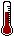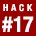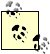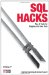# Hack 17. Solve AnagramsYou can use SQL to solve an anagram if you load a dictionary and calculate some hashes.

You can use a hash function to find solutions to certain kinds of word puzzles. In this hack, you will load a dictionary into SQL, tidy it up, and then attach a hash function to every word. With the right hash function you will find that all anagrams hash to the same value. For example, if rat hashes to the number 327, tar will give the same hash value. You can find all of the anagrams of rat by looking in the hash bucket numbered 327.

You can create a table to hold both the words (in a column named w) and the hash value (h). You'll need a type with a large number of bits for h: MySQL has BIGINT which, at 64 bits, is just big enough. Having an index on the hash value makes a big difference; an index on w is handy:

```CREATE TABLE dict
(w VARCHAR(50)
,h BIGINT
,INDEX(w)
,INDEX(h)
);```

MySQL, PostgreSQL, and SQL Server support a 64-bit BIGINT data type. In Oracle, the ROWID data type has 64 bits.

To load a dictionary into your database, you can use the technique shown in "Solve a Crossword Puzzle Using SQL" [Hack #5]. Another method is to use LOAD DATA in MySQL. You can load the file into a temporary table for a little processing before putting the data into dict:

```mysql> CREATE TEMPORARY TABLE tmp(w VARCHAR(50), INDEX(w));
Query OK, 0 rows affected (0.00 sec)

mysql> LOAD DATA LOCAL INFILE '/usr/share/dict/words' INTO TABLE tmp(w);
Query OK, 483523 rows affected (3.87 sec)
Records: 483523 Deleted: 0 Skipped: 0 Warnings: 0```If you don't have the words file on your system (Mac OS X and many Unix and Linux systems do have it), you can obtain word lists from sources such as Moby Word Lists by Grady Ward, available at http://www.gutenberg.org/etext/3201.

This list includes hyphens and apostrophes as well as some uppercase letters. You can remove these characters and force everything into lowercase:

```mysql> UPDATE tmp SET w = REPLACE(REPLACE(LOWER(w),'''',''),'-','');
Query OK, 127204 rows affected (12.13 sec)
Rows matched: 483523 Changed: 127204 Warnings: 0```

That operation introduced a few duplicates, so it would have failed if tmp had a primary key. For example, the tmp table now has two identical rows with the word semicolon because the original list included both semi-colon and semicolon. There is no SQL command that will delete one of those rows but not the other. Here's one way to copy the data without the duplicates:

```mysql> INSERT INTO dict(w) SELECT DISTINCT w FROM tmp;
Query OK, 456402 rows affected (7.20 sec)
Records: 456402 Duplicates: 0 Warnings: 0```

3.3.1. Choose a Hash Function

Now you have to choose a hash function. The hash functions that you normally use, such as MD5, will not do here; you need a hash function that is insensitive to permutation. MD5 would give rat a value different from tar, and you don't want that.

The first hash function that comes to mind is "sum the ASCII codes for each character." With this algorithm, the hash value for rat is 327. All anagrams of rat, such as tar, will have the same hash value, so if you have a list of all the words that hash to 327, you have a list of all the anagrams. There may be a few nonanagrams that happen to have the same hash value, so you should expect some false positives.

That's the theory; now try it.

You need a table of integers [Hack #82] containing the numbers 1 up to the length of the longest word in the list; 64 should be plenty. In this example, the table is called integers, with integer column i.

3.3.1.1. A linear hash function

You can now calculate the sum of the character codes with an UPDATE statement. The ORD function returns the ASCII code for a single character:

```mysql> UPDATE dict
-> SET h = (SELECT SUM(ORD(SUBSTRING(w,i,1)))
-> FROM integers
-> WHERE i <= LENGTH(w));
Query OK, 456402 rows affected (1 min 10.48 sec)
Rows matched: 456402 Changed: 456402 Warnings: 0```

Now you can check the hash value for rat:

```mysql> SELECT * FROM dict WHERE w='rat';
+------+------+
| w | h |
+------+------+
| rat | 327 |
+------+------+```

You use that value, 327, to find all the anagrams of rat:

```mysql> SELECT * FROM dict WHERE h = 327;
+------+------+
| w | h |
+------+------+
| amy | 327 |
| aow | 327 |
| art | 327 |
...
| yam | 327 |
| yma | 327 |
+------+------+
126 rows in set (0.01 sec)```

Whoa! The anagrams of rat (such as art) are certainly on the list, but there are too many false-positive matches, such as yam and amy. You are going to need a better hash function.

Taking the square of each ASCII value generated and then summing the squares should improve the hash distribution. If you square the ASCII values returned from ORD, the numbers generated will be more widely distributed:

```mysql> UPDATE dict
-> SET h = (SELECT SUM(ORD(SUBSTRING(w, i, 1))
-> * ORD(SUBSTRING(w, i, 1)))
-> FROM integers
-> WHERE <= LENGTH(w));
Query OK, 483523 rows affected (1 min 20.50 sec)
Rows matched: 483523 Changed: 483523 Warnings: 0```

You can use a self-join to look up the hash value for a given word:

```mysql> SELECT a.w, a.h FROM dict a
-> JOIN dict b ON (a.h = b.h AND b.w='rat');
+------+-------+
| w | h |
+------+-------+
| art | 35861 |
| atr | 35861 |
| rat | 35861 |
| rta | 35861 |
| tar | 35861 |
| tra | 35861 |
+------+-------+```

There are no false positives in this list, but a longer word shows that there is still a problem:

```mysql> SELECT a.w, a.h FROM dict a JOIN dict b ON (a.h=b.h AND b.w='tango');
+-------+-------+
| w | h |
+-------+-------+
| gonta | 57895 |
| harks | 57895 |
| human | 57895 |
| nahum | 57895 |
| shark | 57895 |
| tango | 57895 |
| tonga | 57895 |
+-------+-------+
7 rows in set (0.00 sec)```

This list is useable. You can pick out the true anagramsgonta and tongafrom the false matches such as harks and human. But you can do better than this, and it does not have to cost any more in terms of processing power.

3.3.1.3. An exponential hash function

Time to get serious! For every letter in each word you add a single bit, where the bit is in position 0 for a, in position 2 for b, 4 for c, and right up to position 50 for z. This allocates two bits for each letter of the alphabet:

```mysql> UPDATE dict
-> SET h = (SELECT SUM(1<<(ORD(SUBSTRING(w,i,1))-97)*2)
-> FROM integers
-> WHERE i<=LENGTH(w));
Query OK, 456402 rows affected (1 min 20.30 sec)
Rows matched: 456402 Changed: 456402 Warnings: 0```

The bit shift operator, <<, is used to move a 1-bit value into the right place. The ORD value of a is 97, so the number of places to shift for character c is (ORD(c)-97)*2.In PostgreSQL, you must change the SUM expression contents: `CAST(1 AS BIGINT)<<(ORD(SUBSTRING(w,i,1))-97)*2`   In SQL Server, you can use: `POWER(CAST(2 AS BIGINT),(ASCII(SUBSTRING(w,i,1))-97)*2)`   In Oracle, you use: `POWER(CAST(2 AS ROWID),(ASCII(SUBSTR(w,i,1))-97)*2)`

Try that out on tango again:

```mysql> SELECT a.w,a.h FROM dict a JOIN dict b ON (a.h=b.h AND b.w='tango');
+-------+--------------+
| w | h |
+-------+--------------+
| gonta | 275213455361 |
| tango | 275213455361 |
| tonga | 275213455361 |
+-------+--------------+
3 rows in set (0.00 sec)```

With this approach, the chance of false positives is significantly reduced. You can see how the hash function works from this result:

```mysql> SELECT w, LPAD(BIN(h),52,0) AS
-> '-z y x w v u t s r q p o n m l k j i h g f e d c b a'
-> FROM dict WHERE w IN ('tango','zoo');
+-------+------------------------------------------------------+
| w | -z y x w v u t s r q p o n m l k j i h g f e d c b a |
+-------+------------------------------------------------------+
| tango | 0000000000000100000000010100000000000001000000000001 |
| zoo | 0100000000000000000000100000000000000000000000000000 |
+-------+------------------------------------------------------+```

In the "tango" line, 01 appears under each letter of the word. In the "zoo" line, 01 is in the z position and 10 is in the o positionthat is 2 in binary for the two o letters.This hashing function will produce false positives only for words where a given letter is repeated more than three times. For example, the string aaaa has a hash value of 4 which is also the hash value for b: `mysql> SELECT a.* FROM dict a JOIN dict b ON (a.h=b.h AND b.w='aaaa');+------+------+| w | h |+------+------+| aaaa | 4 || b | 4 |+------+------+`

If you check the LENGTH of the string as well as the hash value, the chance of a false positive becomes slim:

```mysql> SELECT a.w, a.h FROM dict a
-> JOIN dict b ON (a.h=b.h AND b.w='tango')
-> WHERE LENGTH(a.h) = LENGTH(b.h);
+-------+--------------+
| w | h |
+-------+--------------+
| gonta | 275213455361 |
| tango | 275213455361 |
| tonga | 275213455361 |
+-------+--------------+
3 rows in set (0.00 sec)```SQL Hacks
ISBN: 0596527993
EAN: 2147483647
Year: 2004
Pages: 147